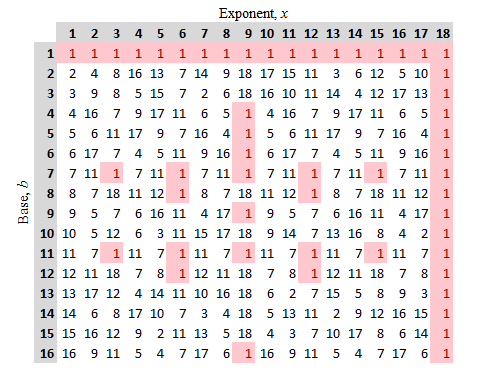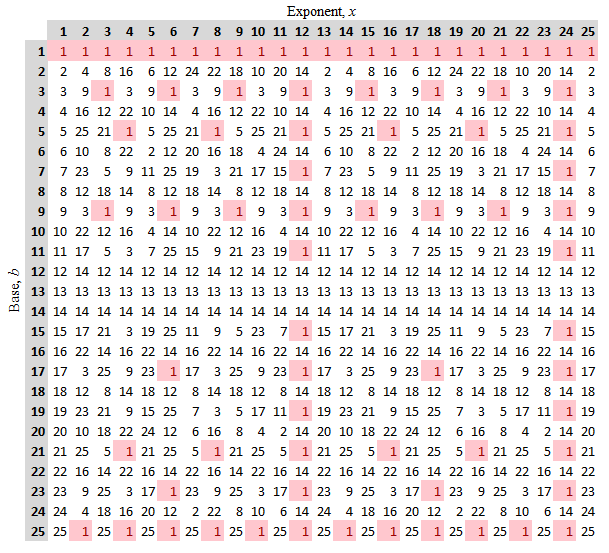## Primitive Roots

Upon looking at the least positive residues of $b^x \pmod{n}$ for various moduli, (as shown for $n=19$ and $n=26$ below), one sees a curious phenomenon when $\textrm{ord}_n b = \varphi(n)$. Namely, the powers $b^x$ seem to cycle through the entire set of the $\varphi(n)$ least positive residues that are relatively prime to the modulus, $n$, with no duplicates.

For example, in the table of powers $\pmod{19}$ below, it appears that the powers of $2$, $3$, $10$, $13$, $14$, and $15$ produce every possible value between $0$ and $19$, exclusive (i.e., the least positive residues that are relatively prime to $19$) -- with no duplicates.Least Positive Residues of $b^x \pmod{19}$

Likewise, in the table of powers $\pmod{26}$ below, $7, 11, 15,$ and $19$ have powers that run through the $\varphi(26) = 12$ least positive residues that are relatively prime to $26$ (i.e., $1$, $3$, $5$, $7$, $9$, $11$, $15$, $17$, $19$, $21$, $23$, and $25$) -- again with no duplicatesLeast Positive Residues of $b^x \pmod{26}$

To attach some verbiage to this idea - let us say, presuming $\gcd(r,n)=1$, and $n \gt 0$, that $r$ is called a primitive root of $n$ when  $\textrm{ord}_n r = \varphi(n)$.

Our conjecture then suggests that if $r$ is a primitive root for some positive integer $n$, then every $r^k$ taken from $$r^1, r^2, r^3, \ldots, r^{\varphi(n)}$$ must be relatively prime to $n$ and no two of these powers can be congruent$\pmod{n}$.

This is relatively straight-forward to prove, however...

First note that if $r$ is a primitive root of $n$, it was presumed that $\gcd(r,n)=1$. So, no prime divisor of $r$ divides $n$. Certainly then, no prime divisor of $r^k$ divides $n$ either, so every $r^k$ is relatively prime to $n$.

Second, suppose that two powers, say $r^i$ and $r^j$, that were congruent modulo $n$. In the discussion on the order of an integer we argued it must then be the case that

$$i \equiv j \pmod{\textrm{ord}_n r}$$

Remember, however, $r$ is a primitive root, so $\textrm{ord}_n r = \varphi(n)$.   Thus,

$$i \equiv j \pmod{\varphi(n)}$$

Since $i$ and $j$ were both positive exponents less than or equal to $\varphi(n)$, it must then be true that

$$i = j$$

Hence, all of the powers $r^1, r^2, r^3, \ldots, r^{\varphi(n)}$ are distinct least positive residues $\pmod{n}$, and are all relatively prime to $n$.

QED.

Interestingly, one can also show that a positive integer, $n \gt 1$, has a primitive root if and only if $n$ takes one of the following forms:

$$n = 2, 4, p^k, \quad \textrm{or} \quad 2p^k,$$

where $p$ is an odd prime and $k$ is a positive integer.

The proof of this fact, however, is considerably less obvious than the one encountered above.